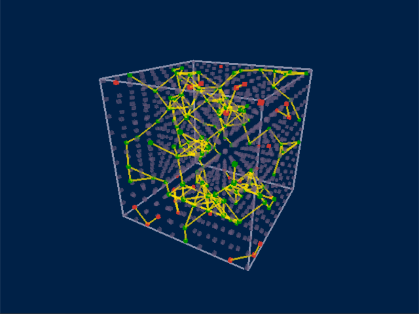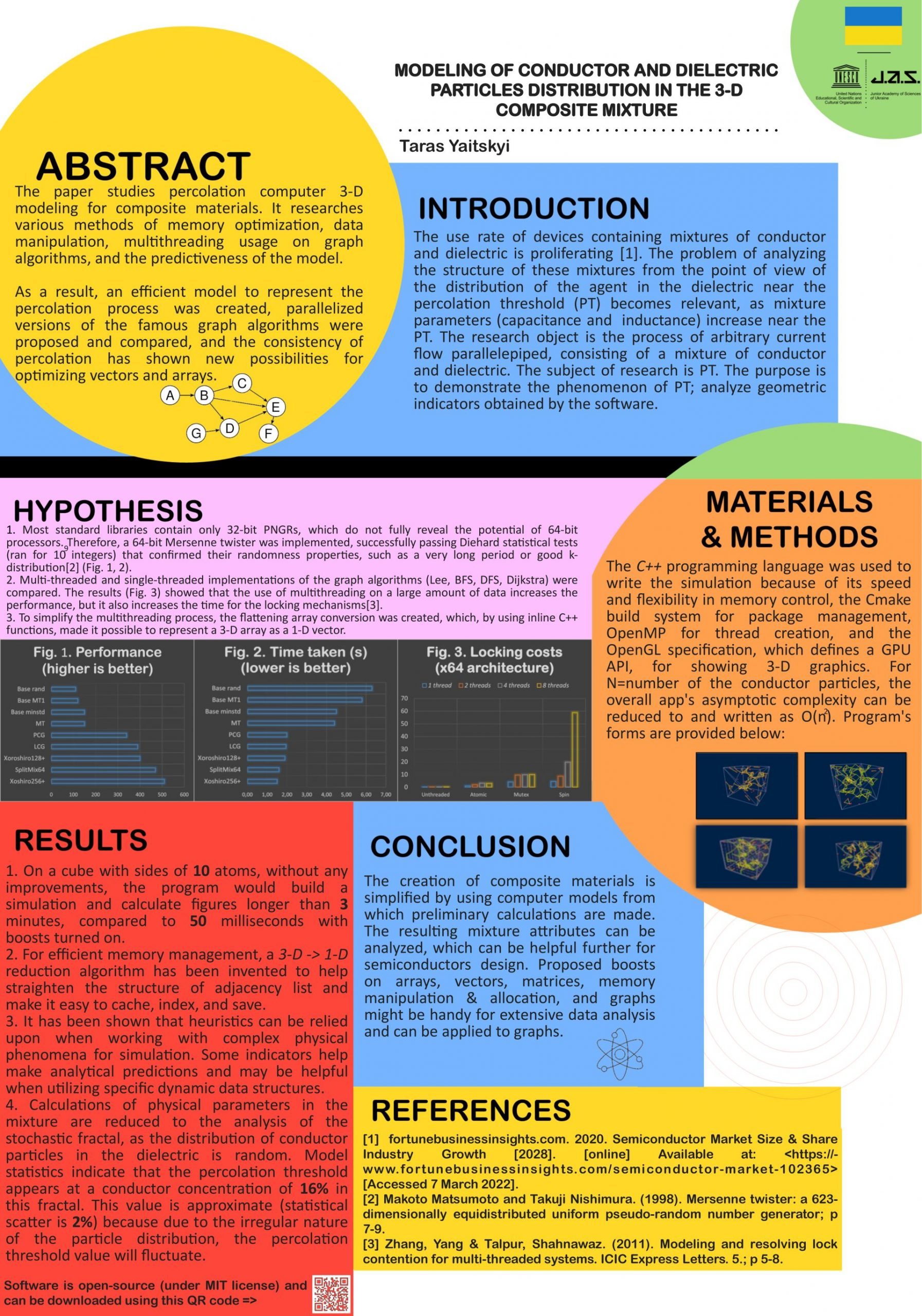# Modeling of conductor and dielectric particles distribution in the 3-D composite mixture

••Author: TARAS YAITSKYI

Menthor: JOSEPH ENTIN

School: UNESCO Centre “Junior Academy of Science of Ukraine” (JASU)

The use rate of devices containing mixtures of conductor and dielectric is proliferating. The production of these devices from other materials requires high costs because the conductor is much more expensive than the dielectric. Thus, the problem of analyzing the structure of these mixtures from the point of view of the distribution of the agent in the dielectric near the percolation threshold (PT) becomes relevant. Some mixture parameters (capacitance and inductance) increase near the PT, which makes the task-relevant, as it becomes possible to reduce the cost of composite materials.

The research object is the process of arbitrary current flow parallelepiped, consisting of a mixture of conductor particles and dielectric. The subject of research is PT. The purpose is to demonstrate the phenomenon of PT, build a computer model, and analyze geometric indicators obtained by the software.

Using the presented algorithms, an efficient model which calculates various parameters (PT was found to be ~16%) of a mixture was created. A revolutionary  array conversion has been designed, and multi-threaded implementations of known algorithms on graphs have been made. Optimizations have accelerated the physics model tenfold, making it possible for real-time rendering.

The creation of composite materials is simplified by using computer models from which preliminary calculations are made. The resulting mixture attributes can be analyzed, which can be helpful further for semiconductors design. Proposed boosts on arrays, vectors, matrices, memory manipulation & allocation, and graphs might be handy for extensive data analysis and applied to graphs.